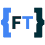## Thursday, October 21, 2010

### Before taking a dip into haskell

I have been itching to start learning another language. I have been perusing through rather a voluminous opinions on what language to learn, on the net.
Too many opinions and it could freeze you from doing something. In any case, I have taken the plunge and would start learning haskell, keeping a commentary on the same here.

Before I do that, I really wanted to have Haskell syntax highlighting support in blogger.

I am yet to test it though. so here is a snippet attached that should have been highlighted. Of course, this code is not mine and just serves to confirm that highlighting works.

module Main where

main = putStrLn "Hello, World!"



I then setup haskell compiler ghc (glasgow haskell compiler) on my mac, using macports.

sudo port install ghc
sudo port install hugs98


I guess, I am ready to dive in.

I have started reading "Programming in Haskell by Graham Hutton" as this seems to be the most recommended book on Haskell introduction.
I am already amazed by the introduction and clarity of differentiation that it brings about between an imperative and functional style of programming. I have to put it here.

Problem # Sum of 0..n numbers

Imperative style:

count := 0
total := 0
repeat
count := count + 1
total := total + count
until count = n


In python

count = 0
total = 0
n = 5
while (count < n):
count = count +1
total = total + count


Functional style:

sum[] =0
sum (x :xs) = x +sum xs

Just few pages and I feel this book is a must read.

A few introductory exercises in the book:

Problem #2 : Define a function product that produces the product of a list of numbers, and show using your definition that product [ 2, 3, 4 ] = 24.

product[ ] = 1
product (x :xs) = x  * product xs

product [ 2, 3, 4 ]            = 2 * product [ 3, 4 ]
= 2 * 3 * product [ 4 ]
= 2 * 3 * 4 * product [ ]
= 2 * 3 * 4 * 1
= 24


Problem #3 : How should the definition of the function qsort be modified so that it produces a reverse sorted version of a list?

qsort[] = []
qsort (x : xs) = qsort larger ++ [x ] ++ qsort smaller
where
smaller = [a | a ← xs, a ≤ x ]
larger = [ b | b ← xs , b > x ]


1.2.